• 对于此练习，我们将使用逻辑回归来识别手写数字（0到9）。 我们将扩展我们在练习2中写的逻辑回归的实现，并将其应用于一对一的分类。 让我们开始加载数据集。 它是在MATLAB的本机格式，所以要加载它在Python，我们...

编程作业 3 - 多类分类

对于此练习，我们将使用逻辑回归来识别手写数字（0到9）。 我们将扩展我们在练习2中写的逻辑回归的实现，并将其应用于一对一的分类。 让我们开始加载数据集。 它是在MATLAB的本机格式，所以要加载它在Python，我们需要使用一个SciPy工具。

import numpy as np
import pandas as pd
import matplotlib.pyplot as plt

data

{'__header__': b'MATLAB 5.0 MAT-file, Platform: GLNXA64, Created on: Sun Oct 16 13:09:09 2011',
'__version__': '1.0',
'__globals__': [],
'X': array([[0., 0., 0., ..., 0., 0., 0.],
[0., 0., 0., ..., 0., 0., 0.],
[0., 0., 0., ..., 0., 0., 0.],
...,
[0., 0., 0., ..., 0., 0., 0.],
[0., 0., 0., ..., 0., 0., 0.],
[0., 0., 0., ..., 0., 0., 0.]]),
'y': array([,
,
,
...,
[ 9],
[ 9],
[ 9]], dtype=uint8)}

data['X'].shape, data['y'].shape

((5000, 400), (5000, 1))

好的，我们已经加载了我们的数据。图像在martix X中表示为400维向量（其中有5,000个）。 400维“特征”是原始20 x 20图像中每个像素的灰度强度。类标签在向量y中作为表示图像中数字的数字类。

第一个任务是将我们的逻辑回归实现修改为完全向量化（即没有“for”循环）。这是因为向量化代码除了简洁外，还能够利用线性代数优化，并且通常比迭代代码快得多。但是，如果从练习2中看到我们的代价函数已经完全向量化实现了，所以我们可以在这里重复使用相同的实现。

sigmoid 函数

g 代表一个常用的逻辑函数（logistic function）为S形函数（Sigmoid function），公式为： $g\left( z \right)=\frac{1}{1+{{e}^{-z}}}$
合起来，我们得到逻辑回归模型的假设函数：
${{h}_{\theta }}\left( x \right)=\frac{1}{1+{{e}^{-{{\theta }^{T}}X}}}$

def sigmoid(z):
return 1 / (1 + np.exp(-z))

代价函数：
J ( θ ) = 1 m ∑ i = 1 m [ − y ( i ) log ⁡ ( h θ ( x ( i ) ) ) − ( 1 − y ( i ) ) log ⁡ ( 1 − h θ ( x ( i ) ) ) ] + λ 2 m ∑ j = 1 n θ j 2 J\left( \theta \right)=\frac{1}{m}\sum\limits_{i=1}^{m}{[-{{y}^{(i)}}\log \left( {{h}_{\theta }}\left( {{x}^{(i)}} \right) \right)-\left( 1-{{y}^{(i)}} \right)\log \left( 1-{{h}_{\theta }}\left( {{x}^{(i)}} \right) \right)]}+\frac{\lambda }{2m}\sum\limits_{j=1}^{n}{\theta _{j}^{2}}

Tip：可以通过np.matrix()函数将一个变量转换为numpy型矩阵

def cost(theta, X, y, learningRate):
# INPUT：参数值theta，数据X，标签y，正则化参数  学习率作业中设为1
# OUTPUT：当前参数值下的交叉熵损失
# TODO：根据参数和输入的数据计算交叉熵损失函数

# STEP1：将theta, X, y转换为numpy类型的矩阵
# your code here  (appro ~ 3 lines)
theta =np.matrix(theta)
X = np.matrix(X)
y = np.matrix(y)

# STEP2：根据公式计算损失函数（不含正则化）
# your code here  (appro ~ 2 lines)

cross_cost =np.multiply(-y, np.log(sigmoid(X * theta.T)))-np.multiply((1 - y), np.log(1 - sigmoid(X * theta.T)))

# STEP3：根据公式计算损失函数中的正则化部分
# your code here  (appro ~ 1 lines)
reg =  (learningRate / (2 * len(X))) * np.sum(np.power(theta[1:], 2))
#reg = (learningRate / (2 * len(X))) * np.sum(np.power(theta[:,1:theta.shape], 2))均可
# STEP4：把上两步当中的结果加起来得到整体损失函数
# your code here  (appro ~ 1 lines)
whole_cost=np.sum(cross_cost)/len(X)+reg   #sum不要忘掉 ，否则最后准确率差别很大

return whole_cost

如果我们要使用梯度下降法令这个代价函数最小化，因为我们未对 θ 0 {{\theta }_{0}} 进行正则化，所以梯度下降算法将分两种情形：
\begin{align}
& Repeat\text{ }until\text{ }convergence\text{ }!!{!!\text{ } \
& \text{ }{{\theta }{0}}:={{\theta }{0}}-a\frac{1}{m}\sum\limits_{i=1}^{m}{[{{h}{\theta }}\left( {{x}^{(i)}} \right)-{{y}{(i)}}]x_{_{0}}{(i)}} \
& \text{ }{{\theta }
{j}}:={{\theta }{j}}-a\frac{1}{m}\sum\limits{i=1}^{m}{[{{h}{\theta }}\left( {{x}^{(i)}} \right)-{{y}{(i)}}]x_{j}{(i)}}+\frac{\lambda }{m}{{\theta }{j}} \
& \text{ }!!}!!\text{ } \
& Repeat \
\end{align}

向量化的梯度函数

# INPUT：参数值theta，数据X，标签y，正则化参数
# OUTPUT：当前参数值下的梯度
# TODO：根据参数和输入的数据计算梯度

# STEP1：将theta, X, y转换为numpy类型的矩阵
# your code here  (appro ~ 3 lines)
theta = np.matrix(theta)
X = np.matrix(X)
y = np.matrix(y)

# STEP2：将theta矩阵拉直（转换为一个向量）
# your code here  (appro ~ 1 lines)
parameters =int(theta.ravel().shape)  #theta数量

# STEP3：计算预测的误差
# your code here  (appro ~ 1 lines)
error = sigmoid(X * theta.T) - y

# STEP4：根据上面的公式计算梯度
# your code here  (appro ~ 1 lines)
grad = ((X.T * error) / len(X)).T + ((learningRate / len(X)) * theta)

# STEP5：由于j=0时不需要正则化，所以这里重置一下
# your code here  (appro ~ 1 lines)
grad[0, 0] = np.sum(np.multiply(error, X[:,0])) / len(X)

现在我们已经定义了代价函数和梯度函数，现在是构建分类器的时候了。 对于这个任务，我们有10个可能的类，并且由于逻辑回归只能一次在2个类之间进行分类，我们需要多类分类的策略。 在本练习中，我们的任务是实现一对一全分类方法，其中具有k个不同类的标签就有k个分类器，每个分类器在“类别 i”和“不是 i”之间决定。 我们将把分类器训练包含在一个函数中，该函数计算10个分类器中的每个分类器的最终权重，并将权重返回为k X（n + 1）数组，其中n是参数数量。

from scipy.optimize import minimize

def one_vs_all(X, y, num_labels, learning_rate):
rows = X.shape
params = X.shape

# k X (n + 1) array for the parameters of each of the k classifiers
all_theta = np.zeros((num_labels, params + 1))

# insert a column of ones at the beginning for the intercept term
X = np.insert(X, 0, values=np.ones(rows), axis=1)  #插了一列1

# labels are 1-indexed instead of 0-indexed
for i in range(1, num_labels + 1):
theta = np.zeros(params + 1)
y_i = np.array([1 if label == i else 0 for label in y])
y_i = np.reshape(y_i, (rows, 1))

# minimize the objective function
fmin = minimize(fun=cost, x0=theta, args=(X, y_i, learning_rate), method='TNC', jac=gradient) # 参数位置保证正确
all_theta[i-1,:] = fmin.x

return all_theta

这里需要注意的几点：首先，我们为theta添加了一个额外的参数（与训练数据一列），以计算截距项（常数项）。 其次，我们将y从类标签转换为每个分类器的二进制值（要么是类i，要么不是类i）。 最后，我们使用SciPy的较新优化API来最小化每个分类器的代价函数。 如果指定的话，API将采用目标函数，初始参数集，优化方法和jacobian（渐变）函数。 然后将优化程序找到的参数分配给参数数组。

实现向量化代码的一个更具挑战性的部分是正确地写入所有的矩阵，保证维度正确。

rows = data['X'].shape
params = data['X'].shape

all_theta = np.zeros((10, params + 1))

X = np.insert(data['X'], 0, values=np.ones(rows), axis=1)

theta = np.zeros(params + 1)

y_0 = np.array([1 if label == 0 else 0 for label in data['y']])
y_0 = np.reshape(y_0, (rows, 1))

X.shape, y_0.shape, theta.shape, all_theta.shape

((5000, 401), (5000, 1), (401,), (10, 401))

注意，theta是一维数组，因此当它被转换为计算梯度的代码中的矩阵时，它变为（1×401）矩阵。 我们还检查y中的类标签，以确保它们看起来像我们想象的一致。

np.unique(data['y'])#看下有几类标签

array([ 1,  2,  3,  4,  5,  6,  7,  8,  9, 10], dtype=uint8)

让我们确保我们的训练函数正确运行，并且得到合理的输出。

all_theta = one_vs_all(data['X'], data['y'], 10, 1)
all_theta

array([[-2.34893352e+00,  0.00000000e+00,  0.00000000e+00, ...,
1.31757773e-03, -3.85035187e-09,  0.00000000e+00],
[-3.16159637e+00,  0.00000000e+00,  0.00000000e+00, ...,
4.38005251e-03, -4.99993633e-04,  0.00000000e+00],
[-4.79720105e+00,  0.00000000e+00,  0.00000000e+00, ...,
-2.86616687e-05, -2.45161016e-07,  0.00000000e+00],
...,
[-7.98140404e+00,  0.00000000e+00,  0.00000000e+00, ...,
-1.00807641e-04,  7.78771593e-06,  0.00000000e+00],
[-4.57449090e+00,  0.00000000e+00,  0.00000000e+00, ...,
-1.18847935e-03,  8.79935802e-05,  0.00000000e+00],
[-5.30054826e+00,  0.00000000e+00,  0.00000000e+00, ...,
-1.12938541e-04,  9.28175840e-06,  0.00000000e+00]])

我们现在准备好最后一步 - 使用训练完毕的分类器预测每个图像的标签。 对于这一步，我们将计算每个类的类概率，对于每个训练样本（使用当然的向量化代码），并将输出类标签为具有最高概率的类。

Tip：可以使用np.argmax()函数找到矩阵中指定维度的最大值

def predict_all(X, all_theta):
# INPUT：参数值theta，测试数据X
# OUTPUT：预测值
# TODO：对测试数据进行预测

# STEP1：获取矩阵的维度信息
rows = X.shape
params = X.shape
num_labels = all_theta.shape

# STEP2：把矩阵X加入一行零元素
# your code here  (appro ~ 1 lines)
X = np.insert(X, 0, values=np.ones(rows), axis=1)

# STEP3：把矩阵X和all_theta转换为numpy型矩阵
# your code here  (appro ~ 2 lines)
X = np.matrix(X)
all_theta = np.matrix(all_theta)

# STEP4：计算样本属于每一类的概率
# your code here  (appro ~ 1 lines)
h = sigmoid(X * all_theta.T)

# STEP5：找到每个样本中预测概率最大的值
# your code here  (appro ~ 1 lines)
h_argmax = np.argmax(h, axis=1)

# STEP6：因为我们的数组是零索引的，所以我们需要为真正的标签+1
h_argmax = h_argmax + 1

return h_argmax

现在我们可以使用predict_all函数为每个实例生成类预测，看看我们的分类器是如何工作的。

y_pred = predict_all(data['X'], all_theta)
correct = [1 if a == b else 0 for (a, b) in zip(y_pred, data['y'])]
accuracy = (sum(map(int, correct)) / float(len(correct)))
print ('accuracy = {0}%'.format(accuracy * 100))

accuracy = 94.5%

正确的话accuracy = 94.46% 差一点点也可以在下一个练习中，我们将介绍如何从头开始实现前馈神经网络。

##error case1 accuracy = 77.58% 10% cost 函数写的有问题

##error case2 调用优化算法 维度不匹配 参数位置问题

展开全文python 机器学习
• Python逻辑回归实现多分类

千次阅读 2019-07-11 10:19:59
分类介绍 分类由二分类问题推广而来，我们可以把N分类问题分解为N个2分类问题。下面我们用代码实现一个简单三分类问题，其中y为n行3列的矩阵，其中0表示不属于该类1表示属于该类。代码中用到的矩阵乘法，不会的...

多分类介绍

多分类由二分类问题推广而来，我们可以把N分类问题分解为N个2分类问题。下面我们用代码实现一个简单三分类问题，其中y为n行3列的矩阵，其中0表示不属于该类1表示属于该类。代码中用到的矩阵乘法，不会的同学自行补课。

代码实现

# coding=utf-8
import random
import matplotlib.pyplot as plt
import numpy as np

x, y = [], []
x_test1, x_test2, x_test3 = [], [], []

# 随机生成3种不同分类的点，分别打上标签存在y中
for i in range(0, 20):
x1 = random.random()
x2 = random.random()
if x1 + x2 < 1:
x.append([x1, x2, 1])
x_test1.append([x1, x2])
y.append([1, 0, 0])

x.append([x1 * 2, x2 + 1, 1])
x_test2.append([x1 * 2, x2 + 1])
y.append([0, 1, 0])

if x1 > x2:
x.append([x1 + 1, x2, 1])
x_test3.append([x1 + 1, x2])
y.append([0, 0, 1])

# 将list转换为numpy array
x = np.array(x)
y = np.array(y)

# 学习率
lr = 0.5

w = np.ones((3, 3))
m = len(x)
for i in range(0, 1000):
w_gard = 0
for j in range(0, m):
# sigmoid函数分类，这里用到了矩阵乘法
w_gard += -x[j] * np.atleast_2d(1 / (1 + np.exp(-np.matmul(w, x[j]))) - y[j]).T
w += lr * w_gard / m

# 画点
plt.plot(np.array(x_test1)[:, 0], np.array(x_test1)[:, 1], 'ro')
plt.plot(np.array(x_test2)[:, 0], np.array(x_test2)[:, 1], 'gx')
plt.plot(np.array(x_test3)[:, 0], np.array(x_test3)[:, 1], 'b.')
# 画分类直线
plt.plot([0, 2], [-w / w, -(w + 2 * w) / w], 'r')
plt.plot([0, 2], [-w / w, -(w + 2 * w) / w], 'g')
plt.plot([0, 2], [-w / w, -(w + 2 * w) / w], 'b')

plt.show()

执行结果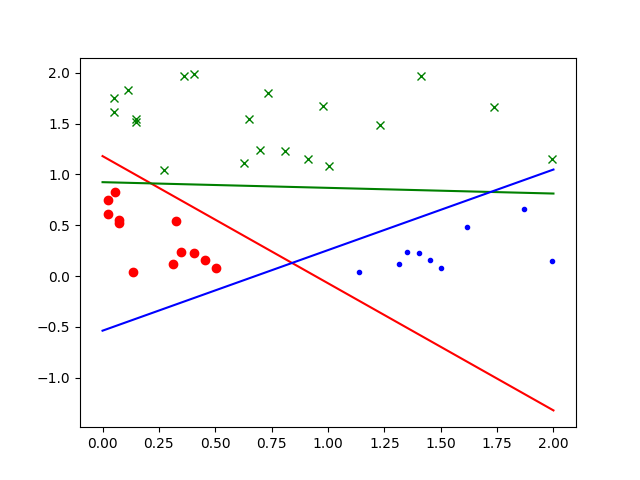我的github上有我的全部学习笔记，欢迎一起学习。github地址：https://github.com/js1219/ML-Learning-Notes.git

展开全文Python 机器学习 逻辑回归 分类
• 前言分类从结果的数量上可以简单的划分为：二分类（Binary Classification）分类（Multinomial Classification）。其中二分类是最常见且使用最多的分类场景，解决二分类的算法有很，比如：基本的KNN、贝叶斯、...

前言

分类从结果的数量上可以简单的划分为：

二分类（Binary Classification）

多分类（Multinomial Classification）。

其中二分类是最常见且使用最多的分类场景，解决二分类的算法有很多，比如：

基本的KNN、贝叶斯、SVM

Online Ranking中用来做二分类的包括FM、FFM、GBDT、LR、XGBoost等

多分类中比如：

改进版的KNN、改进版的贝叶斯、改进版的SVM等

多类别的逻辑回归

啰嗦了这么多，其实就是为了说这个多分类的逻辑回归。

简介

在统计学里，多类别逻辑回归是一个将逻辑回归一般化成多类别问题得到的分类方法。用更加专业的话来说，它就是一个用来预测一个具有类别分布的因变量不同可能结果的概率的模型。

另外，多类别逻辑回归也有很多其它的名字，包括 polytomous LR，multiclass LR，softmax regression，multinomial logit，maximum entropy classifier，conditional maximum entropy model 。

在多类别逻辑回归中，因变量是根据一系列自变量（就是我们所说的特征、观测变量）来预测得到的。具体来说，就是通过将自变量和相应参数进行线性组合之后，使用某种概率模型来计算预测因变量中得到某个结果的概率，而自变量对应的参数是通过训练数据计算得到的，有时我们将这些参数成为回归系数。

模型分析

1、线性分类器

多分类逻辑回归使用的是跟线性回归一致的线性预测函数，其基本表达式如下：

其中i=[1,n]这里的k是一个回归系数，它表示的是第n个观测变量/特征对地n个结果的影响有多大。这里将1看做x0，我们可以得到上述公式的向量化形式：

这里kn是一个回归系数向量，表示的是观测向量x i 表示的观测数据对结果k的影响度，或者叫重要性。

2、逻辑回归

多分类逻辑回归是基于逻辑回归（Logistic Regression）来做的，逻辑回归的基本表示如下：

其中y=1时，f（x）的表达式为：

则y=0时，f（x）的表达式为：

3、k-1个独立二元逻辑回归到多分类逻辑回归的扩展

实现多类别逻辑回归模型最简单的方法是，对于所有K个可能的分类结果，我们运行K−1个独立二元逻辑回归模型，在运行过程中把其中一个类别看成是主类别，然后将其它K−1个类别和我们所选择的主类别分别进行回归。通过这样的方式，如果选择结果K作为主类别的话，我们可以得到以下公式。

其推导过程如下：

这里有个假设的前提：y=K-1和y=K的概率和为1，即：

将逻辑回归的表达式带入可得：

公式两边同时求ln，可得：

在公式（6）中已经引入了所有可能的回归系数集合，对公式（6）两边进行指数化处理，能够得到以下公式：

因为所有概率的和为1，所以可以得到：

这样我们就能计算出所有给定未预测样本情况下某个结果的概率，如下：

回归参数的估计

上面篇幅所涉及到的每一个权重向量 kn 中的未知系数我们可以通过最大后验估计（MAP）来计算，同时也可以使用其它方法来计算，例如一些基于梯度的算法。

二元逻辑回归对数模型到多分类逻辑回归的扩展

在上文中提到了由K-1个独立二元回归到多分类逻辑回归的扩展，这里介绍另外一种多分类逻辑回归的扩展——使用线性预测器和额外的归一化因子（一个配分函数的对数形式）来对某个结果的概率的对数进行建模。

这里用一个额外项 -ln(Z) 来确保所有概率能够形成一个概率分布，从而使得这些概率的和等于1。

然后将等式两边的进行指数化，我们可以得到以下公式：

由于上面说到，所有概率之和等于1，因此我们可以得到 Z 的推导公式：

通过上边的公式进行计算，可得：

综合以上的公式，我们最后可以得到每一个结果对应的概率公式：

仔细观察的话，我们可以发现，所有的概率都具有以下形式：

我们可以把具有以下形式的函数成为softMax函数：

这个函数能够将 x 1 ,...,x n 之间的差别放大，当存在一个 x k 比所有值中的最大值要小很多的话，那么它对应的softMax函数值就会区域0。相反，当 x k 是最大值的时候，除非第二大的值跟它很接近，否则的话softMax会趋于1。所以softmax函数可以构造出一个像是平滑函数一样的加权平均函数。

所以，我们可以把上面的概率公式写成如下softMax函数的形式：

至此，多分类的逻辑回归形式以及介绍完了，后续会进行最大似然函数的学习，敬请期待！

扫描关注【数据与算法联盟】，后台回复【 笔记 】，获得吴恩达老师的机器学习和深度学习的课程笔记。

其他推荐 ：

展开全文• 函数的时候，我们管这样的机器学习算法叫做逻辑回归。 本文源码地址： 关注微信公众号datayx 然后回复“ 逻辑回归 ”即可获取。 sigmoid 函数 这个函数的特点就是一条S型的定义域在 R 中， 值域在 [0,1] 中的函数 ...向AI转型的程序员都关注了这个号👇👇👇

大数据挖掘DT机器学习  公众号： datayx

在我们进行分类的时，所取样本中的特征值一般都分布在实数域，但是我们想得到的往往是一个在 [0,1] 中的类似概率的值。 或者这么说，为了让特征值之间不会因为相差过大而造成干扰，比如，只有一个特征取值特别大，但是其他取值很小的时候， 我们需要对数据进行归一化。 即我们需要用一个从R 到 [0,1] 的单射来先处理特征值矩阵，然后再进行机器学习。当所用的映射是 sigmoid函数的时候，我们管这样的机器学习算法叫做逻辑回归。

本文源码地址：

关注微信公众号datayx 然后回复“逻辑回归”即可获取。

sigmoid 函数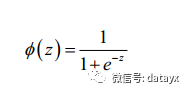这个函数的特点就是一条S型的定义域在 R 中， 值域在 [0,1] 中的函数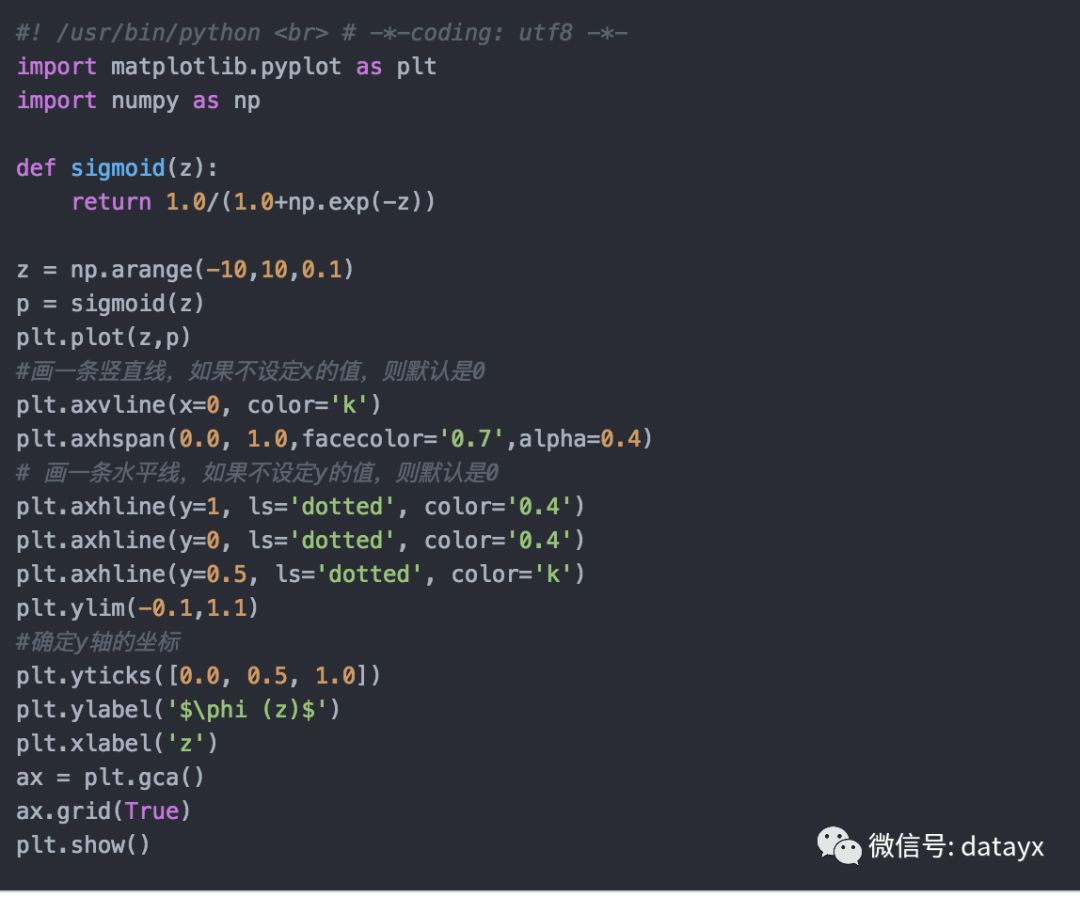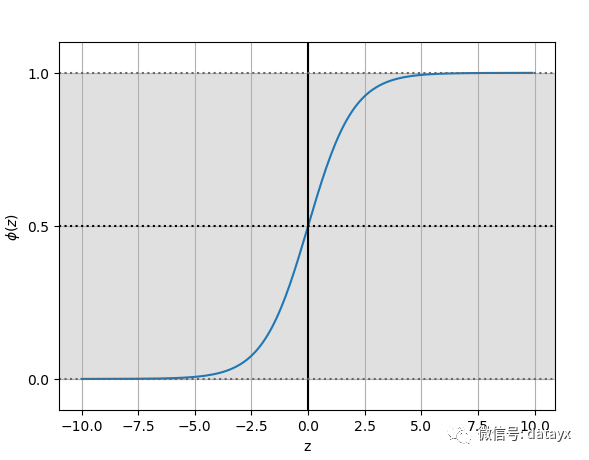逻辑回归算法 logistic regression

• 基本原理Adaline 线性自适应算法很类似，区别只不过是把激活函数从**恒同映射 y = z ** 换成了 y = sigmoid(z)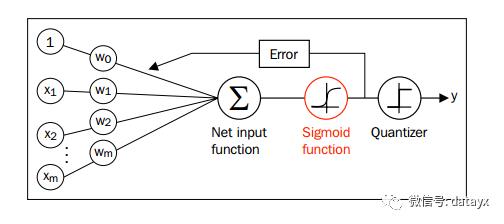• 逻辑回归中的损失函数梯度下降模型 Adaline 中应用到的损失函数 cost function 平方差函数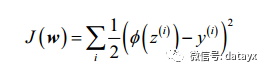logistic regression 损失函数是这样定义的log 其实都是 ln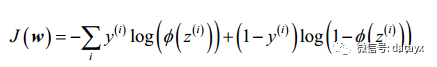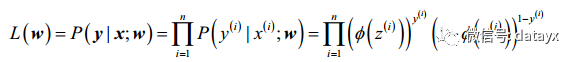http://www.cnblogs.com/kevinGaoblog/archive/2012/03/29/2424346.html

根据似然函数的概念，令似然函数最大的那个概率就是最合理的。我们想最大化似然函数，但是这个形式还是不够好看，毕竟是连乘的形式，所以，我们取一下对数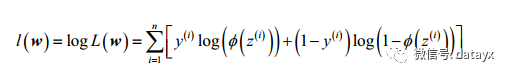w 使 l最大的时候， w 最合理J 函数 ： J = -l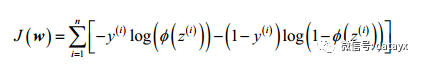为了更好的理解，我们看一下单个样本的损失函数：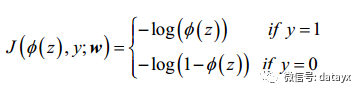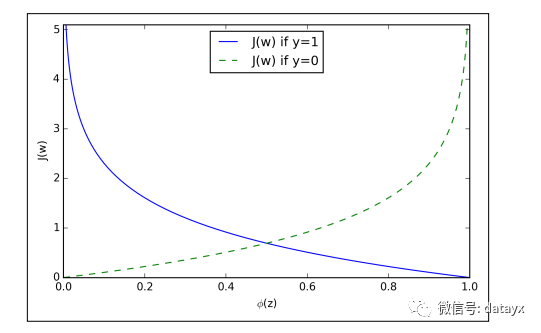J 会收敛到 0

• 权值更新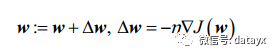经过计算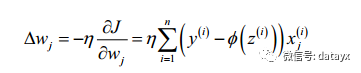我们就有了权值更新的公式

实践

我们再上一章 sklearn 实现 Perceptron 感知机的基础上用 Iris 的数据集来实践一下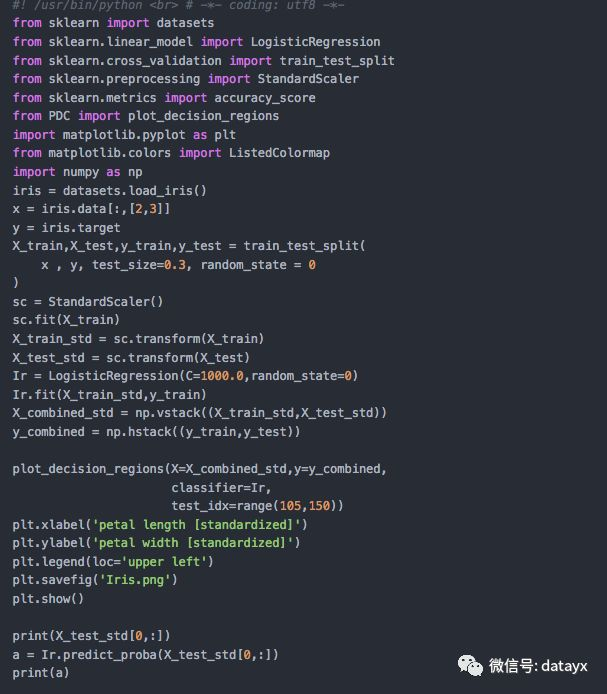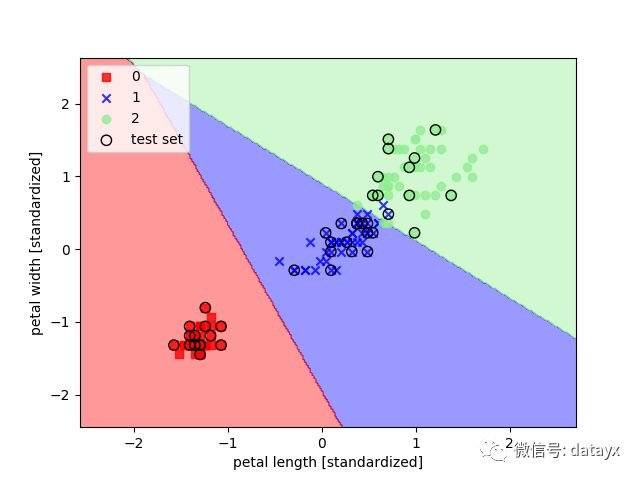过拟合，欠拟合与正则化

过拟合与欠拟合是机器学习常见的两个问题

• 过拟合has a high variance (高方差)

has a high bias (高偏差)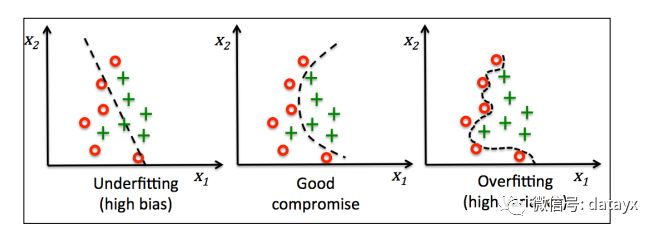• 正则化 RuglarizationL2正则化，他在损失函数的末尾加上这样一项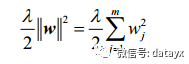Lambda 被称为正则化参数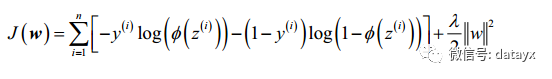Ir = LogisticRegression(C=1000.0,random_state=0)

在类 LogisticRegression 中的参数C 来源于支持向量机(SVM)的相关概念, 这里先不作展开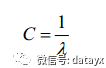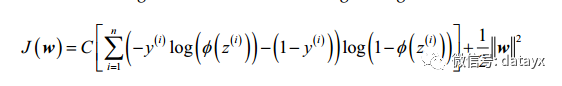• C值对模拟的影响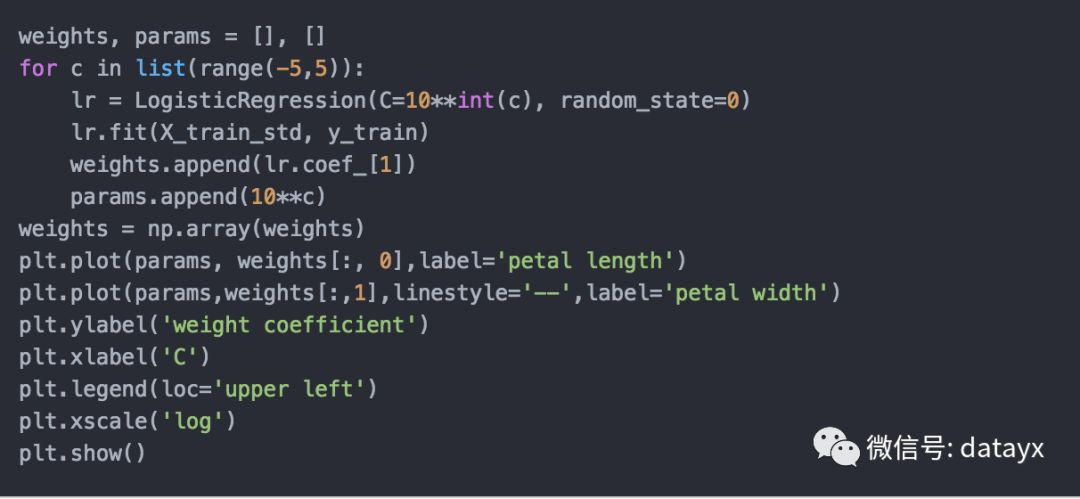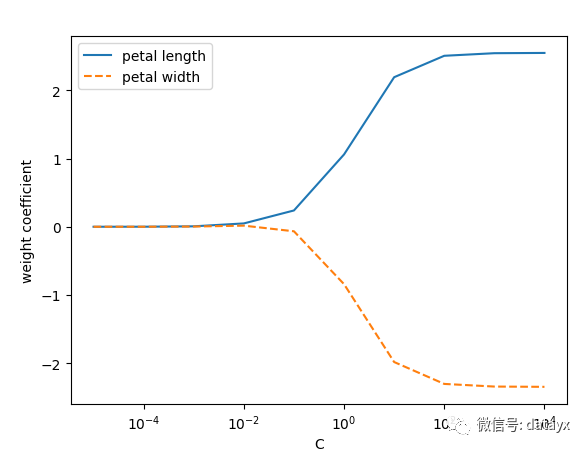垃圾邮件分类

二元分类问题就是垃圾邮件分类（spam classification）。这里，分类垃圾短信。先用TF-IDF算法来抽取短信的特征向量，然后用逻辑回归分类。

数据源：UCI Machine Learning Repository(http://archive.ics.uci.edu/ml/datasets/SMS+Spam+Collection)的短信垃圾分类数据集（SMS SpamClassification Data Set）。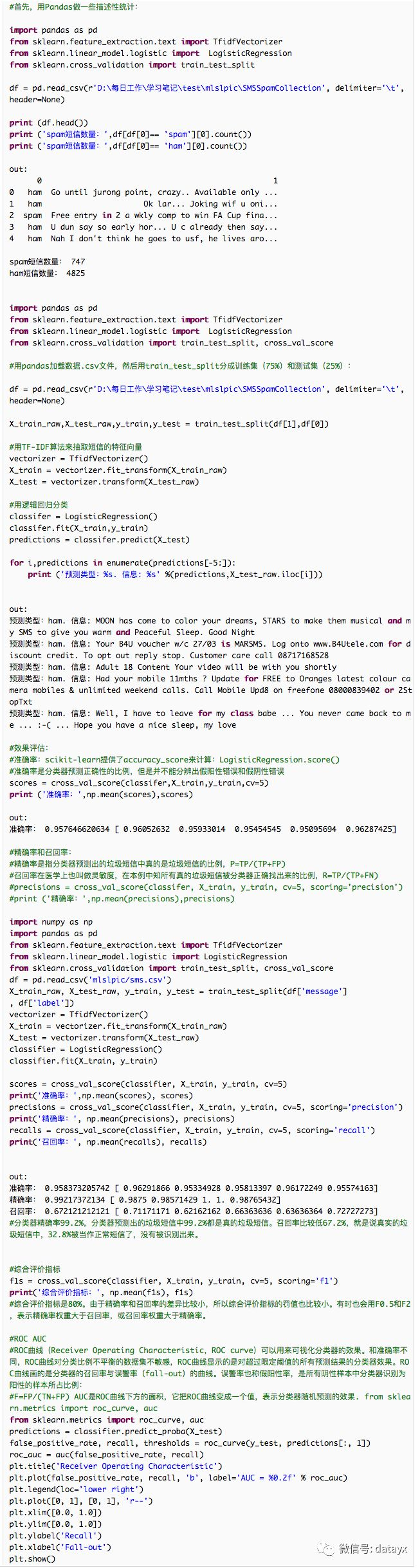网格搜索

网格搜索（Grid search）就是用来确定最优超参数的方法。其原理就是选取可能的参数不断运行模型获取最佳效果。网格搜索用的是穷举法，其缺点在于即使每个超参数的取值范围都很小，计算量也是巨大的。不过这是一个并行问题，参数与参数彼此独立，计算过程不需要同步，所有很多方法都可以解决这个问题。scikit-learn有GridSearchCV()函数解决这个问题：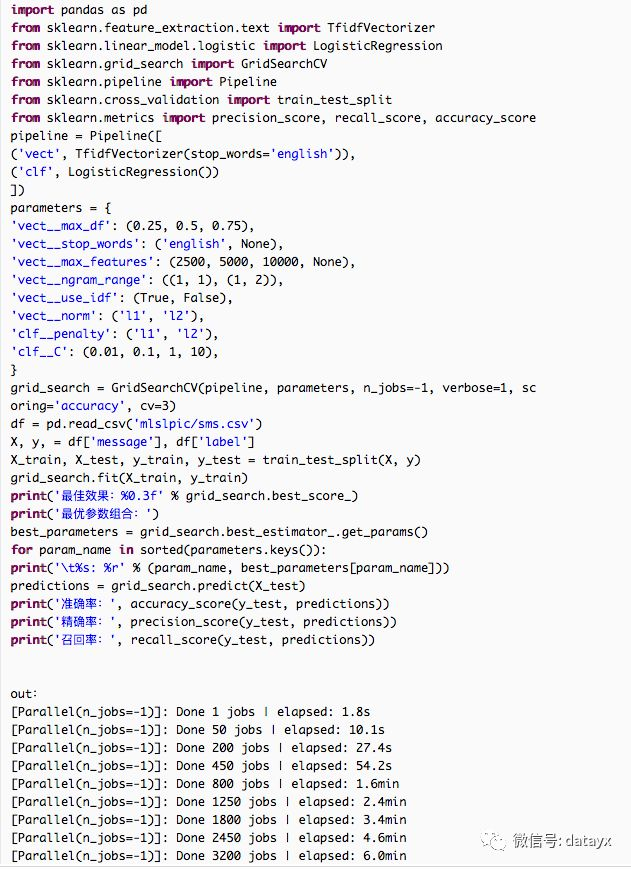GridSearchCV()函数的参数有待评估模型pipeline，超参数词典parameters和效果评价指。

标scoring。n_jobs是指并发进程最大数量，设置为-1表示使用所有CPU核心进程。经过网格计算后的超参数在训练集中取得了很好的效果。

多类分类

scikit-learn用one-vs.-all或one-vs.-the-rest方法实现多类分类，就是把多类中的每个类都作为二元分类处理。分类器预测样本不同类型，将具有最大置信水平的类型作为样本类型。LogisticRegression()通过one-vs.-all策略支持多类分类。

数据集可以从kaggle (https://www.kaggle.com/c/sentiment-analysis-on-movie-reviews)

用烂番茄（Rotten Tomatoes）网站影评短语数据对电影进行评价。每个影评可以归入下面5个类项：不给力（negative），不太给力（somewhat negative），中等（neutral），有点给力（somewhat positive）, 给力（positive）。解释变量不会总是直白的语言，因为影评内容千差万别，有讽刺的，否定的，以及其他语义的表述，语义并不直白。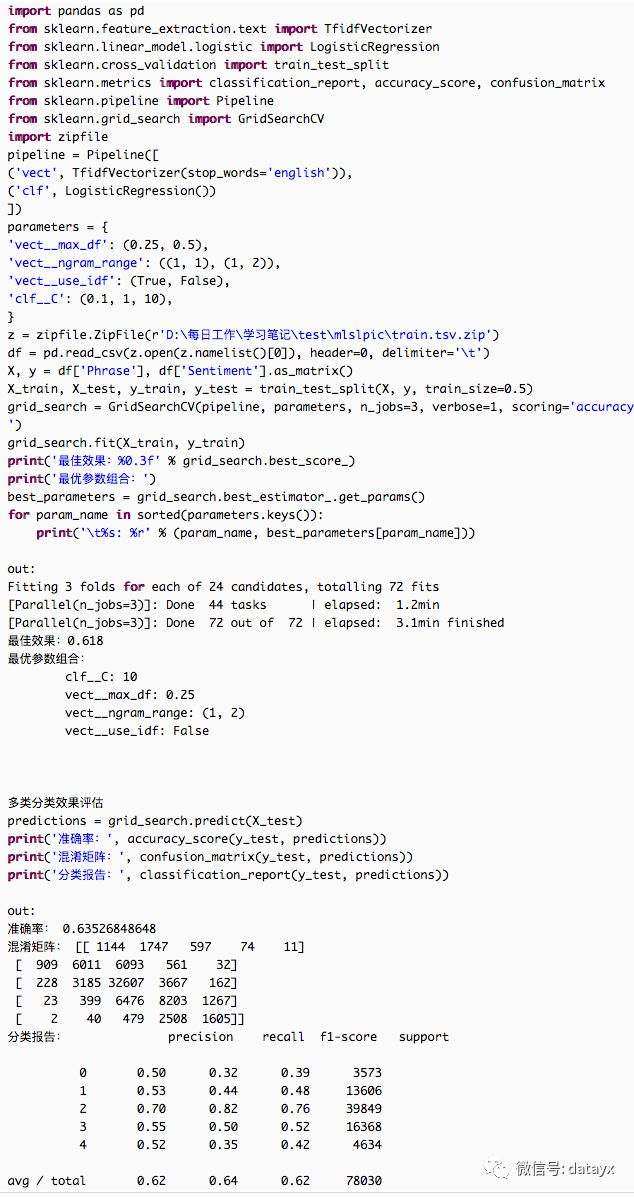多标签分类

多标签分类（multi-label classification）。每个样本可以拥有全部类型的一部分类型。一般有两种解决方法：

问题转化方法(Problem transformation)可以将多标签问题转化成单标签问题。

方法1：训练集里面每个样本通过幂运算转换成单标签。这种幂运算虽然直观，但是并不实用，因为这样做多出来的标

签只有一小部分样本会用到。而且，这些标签只能在训练集里面学习这些类似，在测试集中依然无法使用。

方法2：每个标签都用二元分类处理。每个标签的分类器都预测样本是否属于该标签。这个问题确保了单标签问题和多标签问题有同样的训练集，只是忽略了标签之间的关联关系。

多标签分类效果评估：

最常用的手段是汉明损失函数（Hamming

loss）和杰卡德相似度（Jaccard similarity）。

汉明损失函数表示错误标签的平均比例，是一个函数，当预测全部正确，即没有错误标签时，值为0。

杰卡德相似度或杰卡德相指数（Jaccardindex），是预测标签和真实标签的交集数量除以预测标签和真实标签的并集数量。其值在{0,1}之间，J(Predicted,True)=|Predicted ∩ True|/|Predicted ∪ True|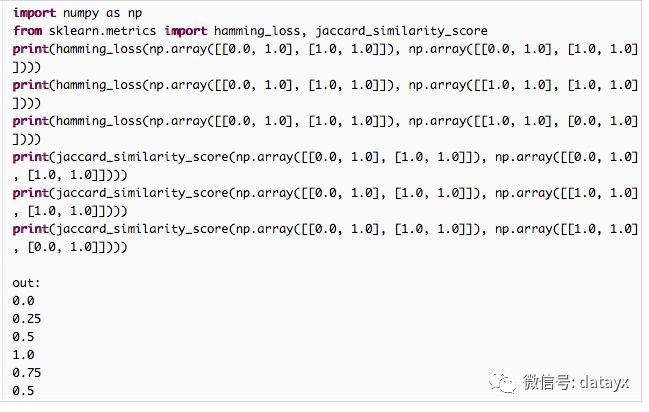搜索公众号添加： datayx

不断更新资源

深度学习、机器学习、数据分析、python长按图片，识别二维码，点关注

展开全文• 题外话——芝诺悖论 只要乌龟在起点拥有些许领先优势的话，兔子将永远追不上乌龟，甚至可以得到乌龟跟兔子无法抵达终点的悖论。来自——《The Math Book》 ...什么是逻辑回归 英文Logistic Regr机器学习 python 逻辑回归机器学习
• 逻辑回归及python代码实现

千次阅读 2020-02-28 03:00:06
逻辑回归可以进行分类操作，但由逻辑回归算法本身性质决定其更常用于二分类。 逻辑回归：线性回归可以预测连续值，但是不能解决分类问题，我们需要根据预测的结果判定其属于正类还是负类。所以逻辑回归就是将线性...python 算法 机器学习 逻辑回归
• 逻辑回归python实现

万次阅读 多人点赞 2018-08-09 10:48:53
这就是逻辑回归要做的事情，并且逻辑回归可以计算出概率 2.模型以及求解（线性） 给出一组m个样本数据，每个样本数据有n个特征，并且带有标记0或者1，代表属于哪一类，为了把输入的参数代入到预测函数后始终是一个...机器学习
• 本文将重点介绍针对类分类问题的逻辑回归的实现。我假设您已经知道如何使用Logistic回归实现二进制分类。如果您尚未使用Logistic回归进行二进制分类，那么建议您先阅读本文，然后再深入研究本文。因为类分类是...
• 一、逻辑回归(LogisticRegression)Logistic regression (逻辑回归)是当前业界比较常用的机器学习方法，用于估计某种事物的可能性。还有类似的某用户购买某商品的可能性，某病人患有某种疾病的可能性啊等等。这个世界...
• Python逻辑回归

2020-12-09 07:22:00
(2)multi_class='multinomal',直接采用分类逻辑回归策略。 12.verbose: 用于开启或者关闭迭代中间输出日志功能。 13.warm_start: 布尔值。如果为True，那么使用前一次训练结果继续训练。否则从头开始训练。 14.n_...
• 文章目录1、逻辑回归简介2、sigmoid函数3、损失函数4、二分类逻辑回归5、分类逻辑回归 1、逻辑回归简介 逻辑回归，实际上不是一个回归算法，而是一个分类算法，应用于对样本数据进行分类的场景中。 逻辑回归的...机器学习 逻辑回归 数据分析
• 逻辑回归一般用来做预测，也可以用来做分类，预测是某个类别^.^！线性回归想比大家都不陌生了，y=kx+b,给定一堆数据点，拟合出k和b的值就行了，下次给定X时，就可以计算出y,这就是回归。而逻辑回归跟这个有点区别，...
• (或者后期有时间了再新写帖子专门记录原理)目录一、python逻辑回归简单案例1. 加载相关库# 基础函数库import numpy as np# 导入画图库import matplotlib.pyplot as pltimport seaborn as sns# 导入逻辑回归模型函数...
• Python机器学习的练习系列共有八个部分：在Python机器学习的练习第3部分中，我们实现了简单的和正则化的逻辑回归。但我们的解决方法有一个限制—它只适用于二进制分类。在本文中，我们将在之前的练习中扩展我们的...
• 逻辑回归同步更新在个人网站：http://www.wangpengcufe.com/machinelearning/pythonml-pythonml6/一、概述1.1、概念是一种名为“回归”的线性分类器，是由线性回归变化而来的，一种广泛使用于分类问题中的广义回归...
• 1.逻辑回归梯度下降的迭代公式 在参考文献1中，我们推导出了逻辑回归的参数...接下来我们按照这个思路，用python来手动实现以下逻辑回归算法。 2.准备数据 -0.017612 14.053064 0 -1.395634 4.662541 1 -0.752...逻辑回归 梯度下降 python
•python 机器学习 数据分析 逻辑回归
• 篇博文主要介绍逻辑回归（logistic regression），首先介绍相关的基础概念和原理，然后通过Python代码实现逻辑回归的二分类问题。特别强调，其中大多理论知识来源于《统计学习方法_李航》和斯坦福课程翻译笔记以及...
• 逻辑回归实现二分类2.1模型训练与预测2.2计算概率2.3绘制决策边界三、逻辑回归实现分类 前言 本篇主要学习逻辑回归模型，使用逻辑回归实现分类任务 。 目标： 能够清晰理解逻辑回归模型的原理。 能够掌握sigmoid...python 机器学习 逻辑回归 可视化
• 正则化逻辑回归-python实现

千次阅读 热门讨论 2021-08-29 15:17:41
正则化逻辑回归-python实现机器学习前言一、基础概念二、构造损失函数三、梯度下降法求解最小值四、正则化逻辑回归总结 基于吴恩达机器学习的习题 逻辑回归github地址 前言 机器学习是从人工智能中产生的一个重要...python 机器学习
• 一般情况下解决分类问题有如下两种方式：1)OvR (One vs Rest)每次将某个与剩下的所有的分类，n个类别进行n次分类，选择分类得分最高的。2). OvO (One vs One)两两组合，比如四个类别有六个组，选择赢数最高的分类...
• 逻辑回归（Logistic Regression）是一种用于解决监督学习（Supervised Learning）问题的学习算法，一般用于二分类（Binary Classification）问题中，用逻辑回归训练得到一个分类器，对输入的数据进行判断其类型，并...逻辑斯蒂回归算法 多分类 数学建模
• 概念针对因变量为分类变量而进行回归分析的一种统计方法，属于概率型非线性回归优点：算法易于实现和部署，执行效率和准确度高缺点：离散型的自变量数据需要通过生成虚拟变量的方式来使用在线性回归中，因变量是连续...
• Python Spark MLlib之逻辑回归

千次阅读 2018-09-11 18:16:43
数据准备 和决策树分类一样，依然使用StumbleUpon Evergreen数据进行实验。 Local模式启动ipython ...PYSPARK_DRIVER_PYTHON=ipython PYSPARK_DRIVER_PYTHON_OPTS="notebook" MASTER=local[*] pyspark...逻辑回归
• 我打算通过逻辑回归来做到这一点(不确定这是否正确，欢迎使用其他方法。)所以这就是我所做的；我对每个工作类别进行了一次k-hot编码(每种工作类型都有1-0值)，而目标i对k-1进行了一次热门编码，并且对Target_ye...
• 手写算法-python代码实现非线性逻辑回归非线性逻辑回归分析用python代码写的逻辑回归类画决策边界 & 用sklearn里面的逻辑回归库画决策边界多项式逻辑回归代码展示总结 非线性逻辑回归分析 上一篇文章，我们介绍...python 机器学习 算法 逻辑回归
• 【火炉炼AI】机器学习009-用逻辑回归分类器解决分类问题(本文所使用的Python库和版本号: Python 3.5, Numpy 1.14, scikit-learn 0.19, matplotlib 2.2 )前面的【火炉炼AI】机器学习008已经讲解了用简单线性分类器...
• 虽然很模型调用一下库就能无脑的使用了，但个人觉得不用框架自己写一次代码的过程还是很有必要的，能让你真正地理解原理与各个步骤的实现过程，而不是停留在貌似懂却连公式都看不懂的阶段。 本文使用softmax回归...机器学习 逻辑回归 人工智能 softmax python
• 逻辑回归的梯度下降算法python实现 ...一、逻辑回归python实现示例代码 import matplotlib.pyplot as plt import numpy as np from sklearn.metrics import classification_report from sklearn im...机器学习 python 逻辑回归 梯度下降算法...

多类别逻辑回归pythonpython 订阅